Latest Banking jobs   »   NIACL AO reasoning

# Reasoning Quiz For NIACL AO Prelims 2023-16th August

Directions (1-4): The question given below is followed by two statements numbered I and II. You have to decide whether the data provided in the statements is sufficient to answer the question:

Q1. Six boxes, M to R are placed one above another, where the bottommost position is 1 and the position above it is 2 & so on. Which box is placed at the bottommost position?
Statements:
I. O is placed 2nd above M which is placed just above of R. One box is placed between N and Q. P is placed below Q.
II. Two boxes are placed between M and N. One box is placed between M and O.

(a) If the data in the statement I alone is sufficient to answer the question, while the data in statement II alone is not sufficient to answer the question.
(b) If the data in the statement II alone is sufficient to answer the question, while the data in statement I alone is not sufficient to answer the question.
(c) If the data either in the statement I alone or in statement II alone is sufficient to answer the question.
(d) If the data in both the statement I and II together are not sufficient to answer the question.
(e) If the data in both the statement I and II together are necessary to answer the question.

Reasoning Quiz For IDBI AM/Executive 2022 16th June CHECK IN HINDI

Q2. There are six members M, N, O, P, Q, and R in the family of three generations. There are couples in each generation of the family. How is Q related to M?
Statements:
I. N is mother-in-law of P who is not married to O. N is not married to R.
II. Q is the wife of O’s father. M is not the father of P who is not in 1st generation.

(a) If the data in the statement I alone is sufficient to answer the question, while the data in statement II alone is not sufficient to answer the question.
(b) If the data in the statement II alone is sufficient to answer the question, while the data in statement I alone is not sufficient to answer the question.
(c) If the data either in the statement I alone or in statement II alone is sufficient to answer the question.
(d) If the data in both the statement I and II together are not sufficient to answer the question.
(e) If the data in both the statement I and II together are necessary to answer the question.

Q3. Five persons X, Y, Z, L and M have different heights. Who among the following is the shortest?
Statements:
I. Y is shorter than only one person. Z is taller than both L and M. X is taller than L.
II. M is taller than only two persons. L is taller than Y but shorter than Z. Y is not the shortest.

(a) If the data in the statement I alone is sufficient to answer the question, while the data in statement II alone is not sufficient to answer the question.
(b) If the data in the statement II alone is sufficient to answer the question, while the data in statement I alone is not sufficient to answer the question.
(c) If the data either in the statement I alone or in statement II alone is sufficient to answer the question.
(d) If the data in both the statement I and II together are not sufficient to answer the question.
(e) If the data in both the statement I and II together are necessary to answer the question.

Q4. Seven persons A, B, C, D, E, F, and G sit in a straight row facing North. Who sit at the extreme left end of the row?
Statements:
I. F sits immediate right of A who sit exactly in the middle of the row. G sits 2nd to the left of B.
II. A sits 3rd to the right of G. Four persons sit between G and D. B sits immediate right of C. F sits to the left of E.

(a) If the data in the statement I alone is sufficient to answer the question, while the data in statement II alone is not sufficient to answer the question.
(b) If the data in the statement II alone is sufficient to answer the question, while the data in statement I alone is not sufficient to answer the question.
(c) If the data either in the statement I alone or in statement II alone is sufficient to answer the question.
(d) If the data in both the statement I and II together are not sufficient to answer the question.
(e) If the data in both the statement I and II together are necessary to answer the question.

Directions (5-8): The question given below is followed by two statements numbered I and II. You have to decide whether the data provided in the statements sufficient to answer the question:

Q5. Eight persons A, B, C, D, E, F, G and H are living in an eight-floor building, not necessarily in the same order. How many persons live below H?
Statements:
I. Only three persons live between E and G who lives either on top or on bottom floor. B and E live on the adjacent floors and B lives three floors above A. Only two persons live between C and D. Minimum three persons live between A and G.
II. D lives four floors above A and two floors below G. Neither D nor A lives on the top and on the bottom floor. E neither lives adjacent to A nor to D. E lives just above C. Only one person lives between B and F.

(a) If the data in the statement I alone is sufficient to answer the question, while the data in statement II alone is not sufficient to answer the question.
(b) If the data in the statement II alone is sufficient to answer the question, while the data in statement I alone is not sufficient to answer the question.
(c) If the data either in the statement I alone or in statement II alone is sufficient to answer the question.
(d) If the data in both the statement I and II together are not sufficient to answer the question.
(e) If the data in both the statement I and II together are necessary to answer the question.

Q6. Point Y is in which direction with respect to point N?
Statements:
I. G is 40 meters west of H. N is 60 meters north of G. H is 52 meters south of J. J is 48 meters east of Y. Y is 20 meters south of K.
II. H is 52 meters east of K. K is 48 meters south of Z. Y is 16 meters north of J. N is 56 meters south of H. J is 20 meters west of Z.

(a) If the data in the statement I alone is sufficient to answer the question, while the data in statement II alone is not sufficient to answer the question.
(b) If the data in the statement II alone is sufficient to answer the question, while the data in statement I alone is not sufficient to answer the question.
(c) If the data either in the statement I alone or in statement II alone is sufficient to answer the question.
(d) If the data in both the statement I and II together are not sufficient to answer the question.
(e) If the data in both the statement I and II together are necessary to answer the question.

Q7. Six persons C, B, A, Z, Y and X belong to a family. Each one of them is having a different age. Who among the following is the youngest in the family?
Statements:
I. C is younger than Y but older than Z. A is older than X. Z is not the youngest among all.
II. Person A, who is 30 years old, is not the oldest. C is 41 years old. X is not the youngest among all.

(a) If the data in the statement I alone is sufficient to answer the question, while the data in statement II alone is not sufficient to answer the question.
(b) If the data in the statement II alone is sufficient to answer the question, while the data in statement I alone is not sufficient to answer the question.
(c) If the data either in the statement I alone or in statement II alone is sufficient to answer the question.
(d) If the data in both the statement I and II together are not sufficient to answer the question.
(e) If the data in both the statement I and II together are necessary to answer the question.

Q8. Seven persons P1, P2, P3, P4, P5, P6 and P7 attend the classes on seven different days starting from Sunday and ending with Saturday, in such a way that one person attends one class in a day, but not necessarily in the same order. Who among the following person attends the class on Saturday?
Statements:
I. Three persons attend the classes between P3 and P7. P5 attends the class just before P3.
II. P1 attends the class on Sunday. One person attends the class between P1 and P4.

(a) If the data in the statement I alone is sufficient to answer the question, while the data in statement II alone is not sufficient to answer the question.
(b) If the data in the statement II alone is sufficient to answer the question, while the data in statement I alone is not sufficient to answer the question.
(c) If the data either in the statement I alone or in statement II alone is sufficient to answer the question.
(d) If the data in both the statement I and II together are not sufficient to answer the question.
(e) If the data in both the statement I and II together are necessary to answer the question.

Directions (9-10): The question given below is followed by two statements numbered I and II. You have to decide whether the data provided in the statements sufficient to answer the question:

Q9. Seven boxes T, U, V, W, X, Y & Z are kept one above other. How many boxes are kept below box U?
Statements:
I. Box X is neither kept adjacent to box Y nor box V. Only three boxes are kept between W and V. Box T is not kept adjacent to box U. Box T is kept at any place above box Z. Box V is kept third from top. Box W and Y are kept together.
II. Box U is neither kept adjacent to box T nor box Y. Box U is kept just below box V. Only three boxes are kept between box W and box V. Only three boxes are kept between box Y and T which is kept second from top.

(a) If the data in the statement I alone is sufficient to answer the question, while the data in statement II alone is not sufficient to answer the question.
(b) If the data in the statement II alone is sufficient to answer the question, while the data in statement I alone is not sufficient to answer the question.
(c) If the data either in the statement I alone or in statement II alone is sufficient to answer the question.
(d) If the data in both the statement I and II together are not sufficient to answer the question.
(e) If the data in both the statement I and II together are necessary to answer the question.

Q10. There are six persons A, B, C, D, E, and F in the family of three generations. Each generation has a couple. Who is the wife of A?
Statements:
I. C is the father-in-law of D. E is married to F. B is the grandmother of E. The gender of B and D is same.
II. A is parent of E who is wife of F. B and C are married.

(a) If the data in the statement I alone is sufficient to answer the question, while the data in statement II alone is not sufficient to answer the question.
(b) If the data in the statement II alone is sufficient to answer the question, while the data in statement I alone is not sufficient to answer the question.
(c) If the data either in the statement I alone or in statement II alone is sufficient to answer the question.
(d) If the data in both the statement I and II together are not sufficient to answer the question.
(e) If the data in both the statement I and II together are necessary to answer the question.

Solutions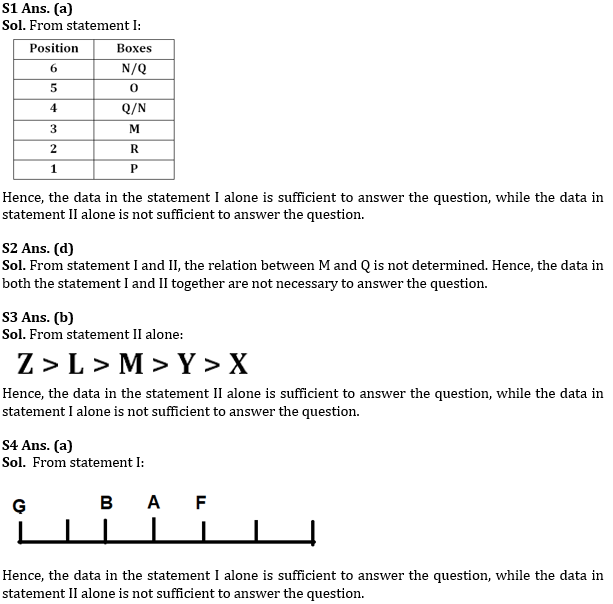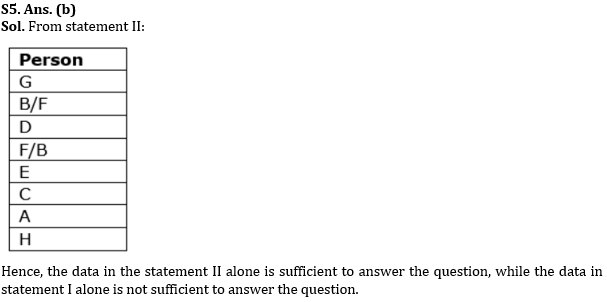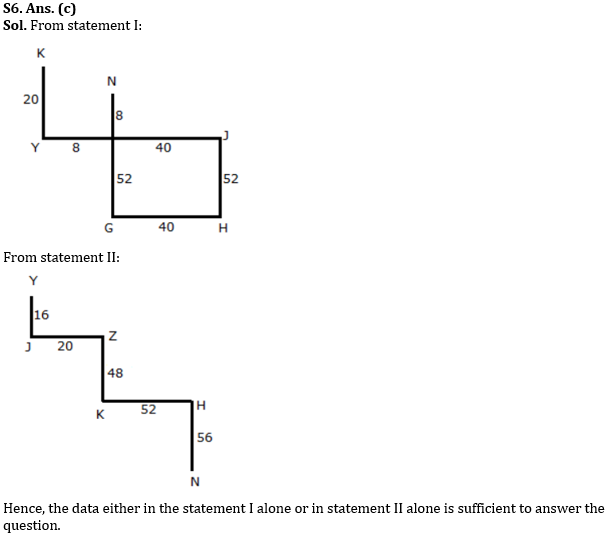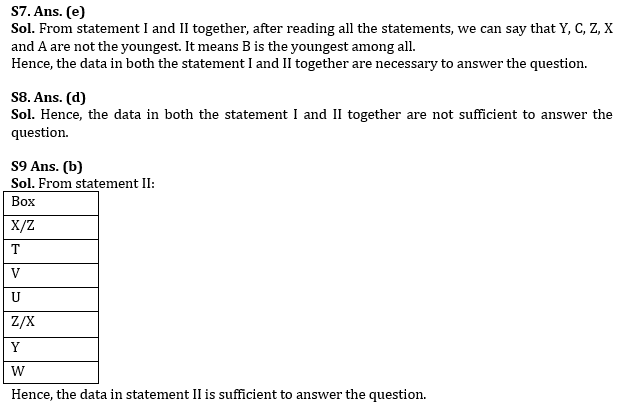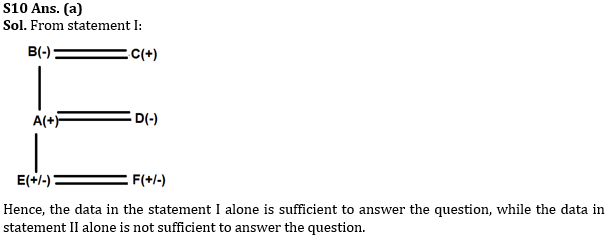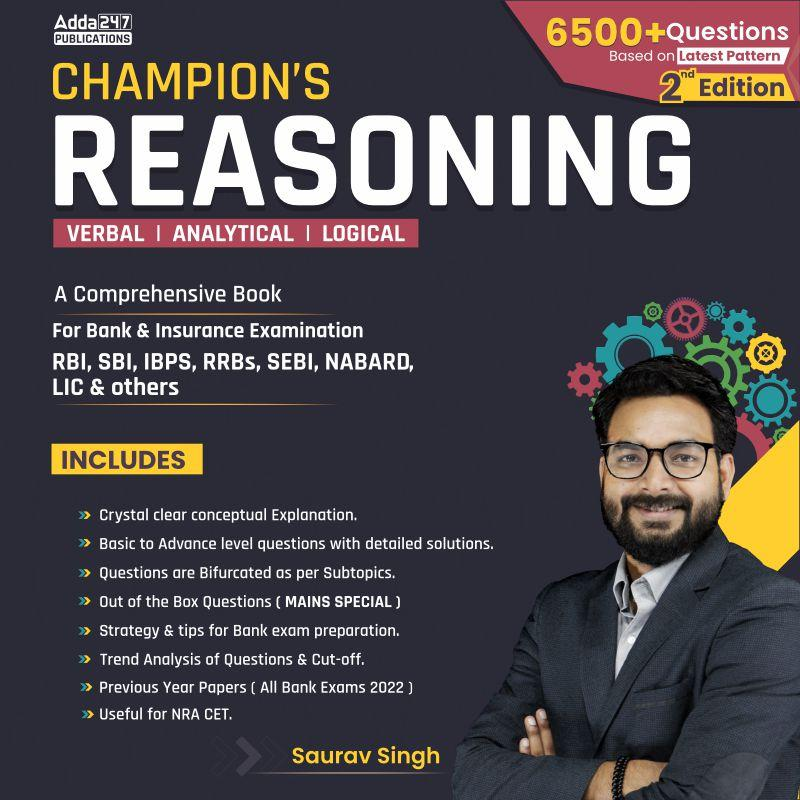## FAQs

### What are the subjects asked in NIACL AO Prelims exam 2023?

Subjects asked in NIACL AO Prelims are Reasoning Ability

#### Congratulations!Union Budget 2023-24: Free PDF